1
44
views
5h
Problem

# Problem 5h

## Chapter 2: Limits and Derivatives

###### Unlmited Homework Help for \$1 and win iPhone 13
Get a \$1 Homework+ and follow @oneclass.store on TikTok for a chance to win an iPhone 13.Textbook ExpertVerified Tutor
13 Oct 2021

#### Given information

Given :- The graph of the function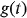is provided below.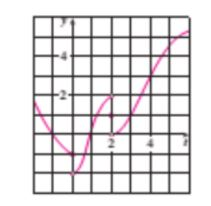To find:- Find the value of the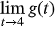.

#### Step-by-step explanation

Step 1.

Apply the concept of one-sided limits

The Left Hand Limit

The limit of a function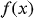is expressed as when the functionis close to a number A when the values of x are sufficiently close to a number b (but less than b).

Mathematically, it is expressed below.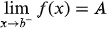As x approaches or tends to from the left, the limit ofis A.

The Right Hand Limit

The limit of a functionis expressed as when the functionis close to a number P when the values of x are sufficiently close to a number b (but greater than b).

Mathematically, it is expressed below.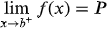As x approaches or tends to from the right, the limit ofis P.